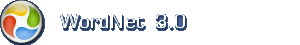# Engelsk ordbog

Info: Dette websted er baseret på WordNet fra Princeton University.

## Engelsk navneord: coefficient

1. coefficient (om mængde el. mål) a constant number that serves as a measure of some property or characteristic

Mindre specifikke termerconstant

Mere specifikke termerabsolute viscosity, absorptance, absorption coefficient, coefficient of absorption, coefficient of drag, coefficient of expansion, coefficient of friction, coefficient of mutual induction, coefficient of reflection, coefficient of self induction, coefficient of viscosity, drag coefficient, dynamic viscosity, expansivity, modulus, mutual inductance, reflectance, reflection factor, reflectivity, self-inductance, transmission, transmittance, weight, weighting